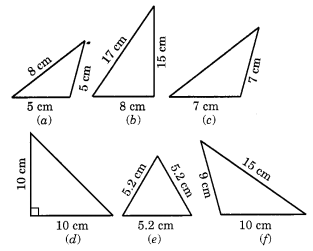## Ex 5.6 Question 1.

Name the types of following triangles:
(а) Triangle with lengths of sides 7 cm, 8 cm and 9 cm.
(b) ∆ABC with AB = 8.7 cm, AC = 7 cm and BC = 6 cm.
(c) ∆PQR such that PQ = QR = PR = 5 cm.
(d) ∆DEF with m∠D = 90°
(e) ∆XYZ with m∠Y = 90° and XY = YZ.
(f) ∆LMN with m∠L = 30° m∠M = 70° and m∠N = 80°.
Solution:
(a) Lengths of the sides of a triangle = 7 cm, 8 cm, and 9 cm.
All sides of the triangle are different.
Hence, it is a Scalene triangle.

(b) In ∆ABC AB = 8.7 cm, AC = 7 cm and BC = 6 cm
All sides of the triangle are different.

Hence, ∆ABC is the Scalene triangle.

(c)In ∆PQR PQ = QR = PR = 5 cm
All sides of the triangle are equal.
Hence, it is an equilateral triangle.

(d) In ∆DEF, m∠D = 90°
Hence it is a right-angled triangle.

(e) In ∆XYZ, m∠Y = 90° and XY = YZ
Hence it is a right-angled triangle.

(f) ∆LMN, m∠L = 30°, m ∠M = 70° and m∠N = 80°.
Hence it is an acute angled triangle.

## Ex 5.6 Question 2.

Match the following:
The measure of triangle                                                     Type of triangle
(i) 3 sides of equal length                                                   (a) Scalene
(ii) 2 sides of equal length                                                  (b) Isosceles right-angled
(iii) All sides are of different length                                   (c) Obtuse angled
(iv) 3 acute angles                                                               (d) Right-angled
(v) 1 right angle                                                                   (e) Equilateral
(vi) 1 obtuse angle                                                               (f) Acute angled
(vii) 1 right angle with two sides of equal length             (g) Isosceles
Solution:
(i) 3 sides of equal length                                                    (e) Equilateral
(ii) 2 sides of equal length                                                   (g) Isosceles
(iii) All sides are of different length                                     (a) Scalene
(iv) 3 acute angles                                                                (f) Acute angled
(v) 1 right angle                                                                   (d) Right-angled
(vi) 1 obtuse angle                                                               (c) Obtuse angled
(vii) 1 right angle with two sides of equal length               (b) Isosceles right-angled

## Ex 5.6 Question 3.

Name each of the following triangles in two different ways: (You may judge the nature of the angle by observation)Solution:

(a) Acute angled and isosceles triangle

(b) Right-angled and scalene triangle

(c) Obtuse angled and isosceles triangle

(d) Right angled and isosceles triangle

(e) Equilateral and acute-angled triangle

(f) Obtuse angled and scalene triangle

Categories: CLASS 6 (MATHS)

error: Content is protected !!